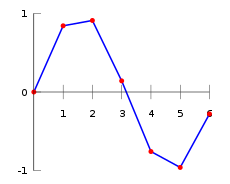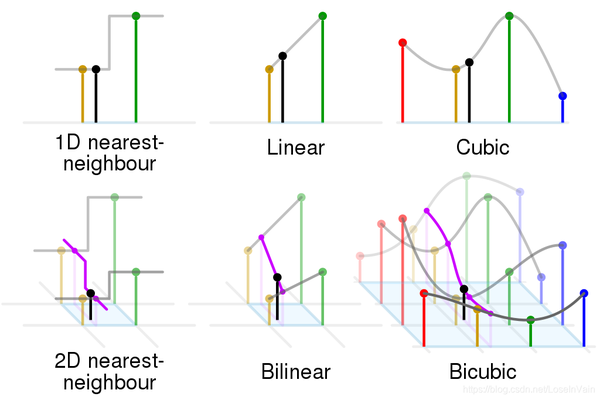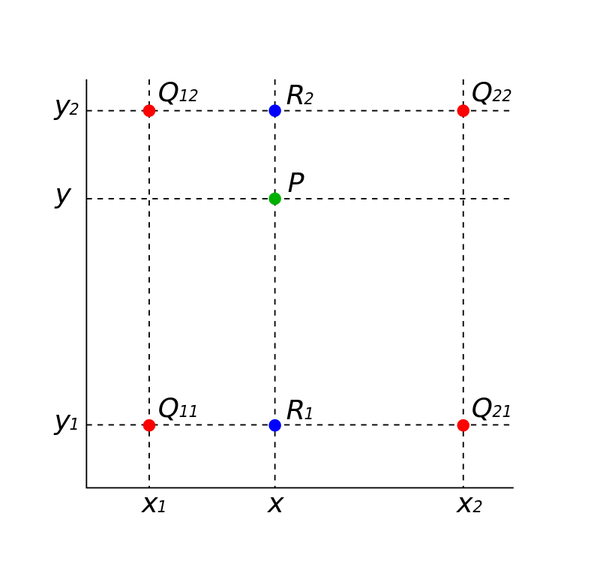# 在 pytorch 中的双线性采样（Bilinear Sample）

FesianXu 2020/09/16 at UESTC

## 前言

$\nabla$ 联系方式：

e-mail: FesianXu\@gmail.com

QQ: 973926198

github: https://github.com/FesianXu

## 双线性插值原理Fig 1.1 一个简单的曲线线性插值的例子。Fig 1.2 常见的1D和2D数据插值方法。

\begin{aligned} Q_{12} &= (x_{1}, y_{2})^{\mathrm{T}} \ Q_{22} &= (x_{2}, y_{2})^{\mathrm{T}} \ Q_{11} &= (x_{1}, y_{1})^{\mathrm{T}} \ Q_{21} &= (x_{2}, y_{1})^{\mathrm{T}} \ \end{aligned} \tag{1.1}Fig 1.3 给定了四个红色数据点（像素点）的值，通过双线性插值求中间的绿色数据点的值。

## 双线性采样以及grid_sample

import torch.nn.functional as F
import torch
inputv = torch.arange(4*4).view(1, 1, 4, 4).float()
print(inputv)
'''

[ 4.,  5.,  6.,  7.],
[ 8.,  9., 10., 11.],
[12., 13., 14., 15.]]]])
'''
# 生成grid，这个grid大小为(1,8,8,2)，空间尺寸而言是原输入图片的两倍。
d = torch.linspace(-1,1, 8)
meshx, meshy = torch.meshgrid((d, d))
grid = torch.stack((meshy, meshx), 2)
grid = grid.unsqueeze(0) # add batch dim

# 进行双线性采样，其中指定align_corners=True保证了输出的整个图片的角边像素与原输入的一致性。
output = F.grid_sample(inputv, grid,align_corners=True)
print(output)
'''
tensor([[[[ 0.0000,  0.4286,  0.8571,  1.2857,  1.7143,  2.1429,  2.5714,
3.0000],
[ 1.7143,  2.1429,  2.5714,  3.0000,  3.4286,  3.8571,  4.2857,
4.7143],
[ 3.4286,  3.8571,  4.2857,  4.7143,  5.1429,  5.5714,  6.0000,
6.4286],
[ 5.1429,  5.5714,  6.0000,  6.4286,  6.8571,  7.2857,  7.7143,
8.1429],
[ 6.8571,  7.2857,  7.7143,  8.1429,  8.5714,  9.0000,  9.4286,
9.8571],
[ 8.5714,  9.0000,  9.4286,  9.8571, 10.2857, 10.7143, 11.1429,
11.5714],
[10.2857, 10.7143, 11.1429, 11.5714, 12.0000, 12.4286, 12.8571,
13.2857],
[12.0000, 12.4286, 12.8571, 13.2857, 13.7143, 14.1429, 14.5714,
15.0000]]]])
'''


$$f{x} = f{y} = \dfrac{3}{2}x_{norm}+\dfrac{3}{2} \tag{1}$$